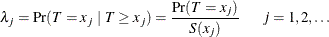### Failure Time Distribution

Let T be a nonnegative random variable representing the failure time of an individual from a homogeneous population. The survival distribution function (also known as the survivor function) of T is written as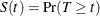A mathematically equivalent way of specifying the distribution of T is through its hazard function. The hazard function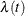specifies the instantaneous failure rate at t. If T is a continuous random variable,is expressed as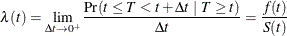where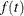is the probability density function of T. If T is discrete with masses at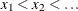, then survivor function is given by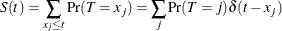where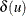=0 if u < 0 and=1 otherwise. The discrete hazards are given by